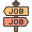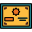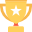# Python for Finance

Python in financial industry is mainly used for quantitative and qualitative analysis. Stock market analysis, predictions, deep learning and machine learning on stocks are mainly done using python. Learn Python for Finance: Investment Fundamentals & Data Analytics from Scratch in 3 months. TryCatch Classes provides the best Python for Finance Course in Mumbai, Thane students. We teach Python from scratch and provide practical classroom training for Python course.

## WHAT YOU WILL LEARN:

• Learn how to code in Python
• Work with Python’s conditional statements, functions, sequences, and loops
• Understand how to use the data analysis toolkit, Pandas
• Use Python to solve real-world tasks
• Acquire solid financial acumen.
• Build investment portfolios.
• Calculate risk and return of investment portfolios.
• Use univariate and multivariate regression analysis.
• Compare securities in terms of their Sharpe ratio.
• Learn how to price options by applying the Black Scholes formula.
• Work with scientific packages, like NumPy.
• Plot graphs with Matplotlib.
• Carry out in-depth investment analysis.
• Calculate risk and return of individual securities.
• Apply best practices when working with financial data
• Understand the Capital Asset Pricing Model
• Perform Monte Carlo simulations
• Be comfortable applying for a developer job in a financial institution

Learn Python Programming and Conduct Real-World Financial Analysis in Python.

## COURSE MODULE:

#### Python for Finance Course Content

Module 1:
Introduction to Python
• What is Python
• Why Python?
• Why Jupyter?
• Installing Python and Jupyter.
• Jupyter’s Interface – the Dashboard
• Jupyter’s Interface – Prerequisites for Coding
Module 2:
Python Variables and Data Types
• Variables.
• Numbers and Boolean Values.
• Strings.
Module 3:
Basic Python Syntax
• Arithmetic Operators.
• The Double Equality Sign.
• Reassign Values.
• Line Continuation.
• Indexing Elements.
• Structure Your Code with Indentation.
• Comparison Operators
• Logical and Identity Operators
Module 4:
Conditional Statements
• Introduction to the IF statement.
• Else if, for Brief – ELIF.
• A Note on Boolean values
Module 5:
Python Functions
• Defining a Function in Python.
• Creating a Function with a Parameter.
• Combining Conditional Statements and Functions.
• Notable Built-in Functions in Python.
Module 6:
Python Sequences
• Lists.
• List Slicing.
• Tuples.
• Sets.
• Dictionaries.
Module 7:
Using Iterations in Python
• For Loops.
• While Loops and Incrementing.
• Create Lists with the range() Function.
• Use Conditional Statements and Loops Together.
• All In – Conditional Statements, Functions, and Loops.
• Iterating over Dictionaries
Module 8:
• Object Oriented Programming.
• Modules and Packages.
• The Standard Library.
• Importing Modules
• Must-have packages for Finance and Data Science.
• Working with arrays
• Generating Random Numbers
• A Note on Using Financial Data in Python
• Sources of Financial Data
• Accessing the Notebook Files
• Importing and Organizing Data in Python
• Changing the Index of Your Time-Series Data
• Restarting the Jupyter Kernel
Module 9:
PART II FINANCE:
Calculating and Comparing Rates of Return in Python
• Considering both risk and return.
• Calculating a security’s rate of return.
• Calculating a Security’s Rate of Return in Python – Simple Returns.
• What is a portfolio of securities and how to calculate its rate of return.
• Popular stock indices that can help us understand financial markets.
Module 10:
PART II Finance:
Measuring Investment Risk
• How do we measure a security’s risk?
• Calculating a Security’s Risk in Python.
• The benefits of portfolio diversification.
• Investing in stocks.
• Calculating the covariance between securities.
• Measuring the correlation between stocks.
• Calculating Covariance and Correlation.
• Considering the risk of multiple securities in a portfolio.
• Understanding Systematic vs. Idiosyncratic risk.
• Calculating Diversifiable and Non-Diversifiable Risk of a Portfolio.
Module 11:
PART II Finance –
Using Regressions for Financial Analysis
• The fundamentals of simple regression analysis.
• Running a Regression in Python.
• Are all regressions created equal? Learning how to distinguish good regressions.
• Computing Alpha, Beta, and R Squared in Python.
Module 12:
PART II Finance –
Markowitz Portfolio Optimization
• Markowitz Portfolio Theory – One of the main pillars of modern Finance.
• Obtaining the Efficient Frontier in Python.
Module 13:
Part II Finance –
The Capital Asset Pricing Model
• The intuition behind the Capital Asset Pricing Model (CAPM).
• Understanding and calculating a security’s Beta.
• Calculating the Beta of a Stock.
• The CAPM formula.
• Calculating the Expected Return of a Stock (CAPM).
• Introducing the Sharpe ratio and how to put it into practice.
• Obtaining the Sharpe ratio in Python.
• Measuring alpha and verifying how good (or bad) a portfolio manager is doing.
Module 14:
Part II Finance:
Multivariate regression analysis
• Multivariate regression analysis – a valuable tool for finance practitioners.
• Running a multivariate regression in Python.
Module 15:
PART II Finance –
Monte Carlo simulations as a decision-making tool
• The essence of Monte Carlo simulations.
• Monte Carlo applied in a Corporate Finance context.
• Monte Carlo: Predicting Gross Profit
• Forecasting Stock Prices with a Monte Carlo Simulation.
• Monte Carlo: Forecasting Stock Prices.
• An Introduction to Derivative Contracts.
• The Black Scholes Formula for Option Pricing.
• Monte Carlo: Black-Scholes-Merton.
• Monte Carlo: Euler Discretization.

#####PLACEMENT GUARANTEE:

We offer 100% placement guarantee in Python for Finance Course and that is why we are the no 1 Python for Finance training classes in Mumbai. At TryCatch Classes, we train students for interviews and offer placements in corporate companies.

#####CERTIFICATION:

At the end of the Python for Finance training course in Mumbai, you will get a Python for Finance Certification from us which will be very helpful for you in your future.

#####AWARDS:

TryCatch Classes has been awarded multiple times as the “Most Promising Software Training Institute in Maharashtra” and “Social Impact Award for Outstanding Contribution to Indian Education System” at Education Leadership Awards.

#### OTHER RELATED COURSES:

TryCatch Classes provides the best  Python for Finance: Investment Fundamentals & Data Analytics